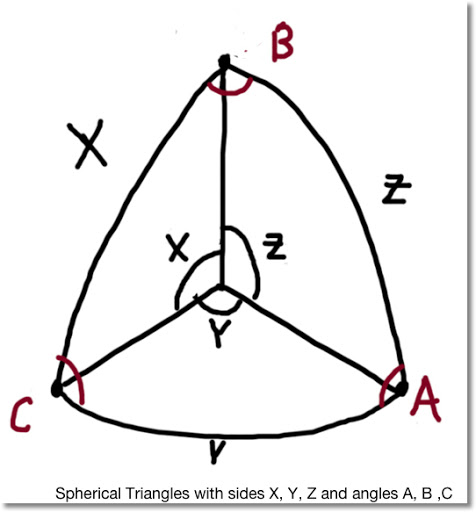## Friday, July 5, 2013

### Spherical Triangle Solver - TI-84+Spherical Triangle Solver: Programmed on a TI-84+ C Silver Edition

This could easily be ported to other programming calculators given how basic the TI-84+ programming is.

Variables:
"Sides" (Subtending Angles): X, Y, Z
Corresponding Angles: A, B, C

I try to capture all possible traps. No guarantees by I try. This program is rather large, about 1,500 bytes. Ending quotes and parenthesis can be left out on the TI-84+ but I present them in the text for completeness.

PROGRAM: SPHTRI
: Lbl 0
: ClrHome
: Output(1,1,"ANGLE (θ),SIDE")
: Output(2,1,"A, X")
: Output(3,1,"B, Y")
: Output(4,1,"C, Z")
: Output(6,1,"PRESS ENTER TO GO ON")
: Pause
: ClrHome
"2 S,INT θ (X,Y,C)",2,
"2 S,EXT θ (Y,Z,B)",3,
"1 S,2 ADJ θ (Z,A,B)",4,
"2 θS, ADJ SIDE (X,A,B)",5,
"3 ANGLES", 6
"EXIT",7)

: Lbl 1
: Input "SIDE X:", X
: Input "SIDE Y:", Y
: Input "SIDE Z:", Z
: If X+Y+Z>360°
: Then
: Disp "NO SOLN"
: Pause
: Goto 0
: End
: cos^-1 (( cos(X) - cos(Y) cos(Z) )/( sin(Y) sin(Z) )→ A
: cos^-1 (( cos(Y) - cos(X) cos(Z) )/( sin(X) sin(Z) )→ B
: cos^-1 (( cos(Z) - cos(X) cos(Y) )/( sin(X) sin (Y) )→ C
: Disp "A,B,C:",A,B,C
: Pause
: Goto 0

: Lbl 2
: Input "SIDE X:", X
: Input "SIDE Y:", Y
: Input "θ BETWEEN C:", C
: cos^-1 ( cos(X) cos(Y) + sin(X) sin(Y) cos(C) ) → Z
: sin^-1 ( sin(C) sin(X) / sin(Z) ) → A
: sin^-1 ( sin(C) sin(Y) / sin(Z) ) → B
: Disp "Z,A,B:",Z,A,B
: Pause
: Goto 0

: Lbl 3
: Input "SIDE Y:", Y
: Input "CORR. θ B:", B
: Input "ADJ. SIDE Z:", Z
: If Y < sin^-1 (sin(Z) sin(B) )
: Then
: Disp "NO SOLN."
: Pause
: Goto 0
: End
: sin^-1 ( sin(Z) sin(B) / sin(Y) ) → C
: 2 tan^-1 ( tan(.5Y - .5Z) sin(.5B + .5C) / sin(.5B - .5C) ) → X
: 2 tan^-1 ( sin(.5Y - .5Z) / tan(.5B - .5C) / sin(.5Y + .5Z) ) → A
: Disp "C,X,A:",C,X,A
: Pause
: If Y: Then
: 180° - C → S
: 2 tan^-1 (tan(.5Y - .5Z) sin(.5B + .5C) / sin(.5B - .5C) ) → T
: 2 tan^-1 (sin(.5Y - .5Z) / tan(.5B - .5S) / sin(.5Y + .5Z) ) → U
: Disp "ALT C,X,A:",S,T,U
: Pause
: End
: Goto 0

: Lbl 4
: Input "θ A:", A
: Input "θ B:", B
: Input "SIDE BETWEEN Z:", Z
: cos^-1 ( sin(A) sin(B) cos(Z) - cos(A) cos(B) ) → C
: cos^-1 ( (cos(A) + cos(B) cos(C)) / (sin(B) sin(C)) → X
: cos^-1 ( (cos(B) + cos(A) cos(C)) / (sin(A) sin(C)) → Y
: Disp "C,X,Y:",C,X,Y
: Pause
: Goto 0

: Lbl 5
: Input "θ A:", A
: Input "CORR. SIDE X:", X
: Input "ADJ θ B:", B
: sin^-1 (sin(X) sin(B) / sin(A) ) → Y
: 2 tan^-1 (tan(.5X-.5Y) sin(.5A+.5B) / sin(.5A-.5B)) → Z
: 2 tan^-1 (sin(.5X-.5Y) / tan(.5A-.5B) / sin(.5X+.5Y)) → C
: Disp "Y,C,Z:", Y,C,Z
: Pause
: Goto 0

: Lbl 6
: Input "θ A:", A
: Input "θ B:", B
: Input "θ C:", C
: If A+B+C<180° or A+B+C>540°
: Then
: Disp "NO SOLN."
: Goto 0
: Pause
: End
: Prompt A,B,C
: cos^-1 ((cos(A) + cos(B) cos(C))/(sin(B) sin(C)) → X
: cos^-1 ((cos(B) + cos(A) cos(C))/(sin(A) sin(C))→ Y
: cos^-1 ((cos(C) + cos(A) cos(B))/(sin(A) sin(B)) → Z
: Disp "X,Y,Z:",X,Y,Z
: Pause
: Goto 0

: Lbl 7

Sources:

Wikipedia: Solutions of Triangles
http://en.wikipedia.org/wiki/Solution_of_triangles#Solving_spherical_triangles

Had To Know: Spherical Trigonometry Calculator

Examples - in Degrees Mode:

1. Given 3 Sides:
X: 34.49°
Y: 28.11°
Z: 29.00°
Solutions:
A: 76.64274255°
B: 54.05239674°
C: 56.40782913°

2. Given 2 Side with Interior Angle
X: 5.58°
Y: 7.24°
C: 164°
Solutions:
Z: 12.69684035°
A: 7.004143971°
B: 9.093527785°

3. Given 2 Sides with Exterior (Adjacent) Angle:
Y: 14.41°
B: 77.97°
Z: 18.00°
No Solution

Y: 7.09°
B: 44.8°
Z: 6.36°
Solutions:
C: 39.22735167°
X: 10.02551866°
A: 96.36477875°

4. Given 1 side and 2 adjacent angles
A: 75°
B: 88°
Z: 4.53°
Solutions:
C: 17.58132838°
X: 14.62939892°
Y: 15.14817154°

5. Given 2 Angles and 1 adjacent side
A: 103°
X: 2.66°
B: 71°
Solutions:
Y: 2.581182188°
C: 6.006268443°
Z: .2855550692°

6. Given 3 interior angles
A: 89°
B: 66°
C: 70°
Solutions:
X: 79.49157643°
Y: 63.94337829°
Z: 67.52897419°

This blog is property of Edward Shore. 2013